# MSBSHSE Solutions For Class 8 Maths Part 2 Chapter 14 Compound interest

MSBSHSE Solutions For Class 8 Maths Part 2 Chapter 14 Compound interest is given here to help students prepare for their examinations at ease. Students facing difficulties in solving problems during class hours can quickly refer to MSBSHSE Solutions to get a better understanding. The facilitators at BYJU’S have developed these solutions, considering the grasping abilities of different students. All the solutions are in accordance with the latest Maharashtra Board guidelines.

This chapter covers topics such as calculating the compound interest for a given sum and duration, finding the amount after a given duration under compound interest and application of the formula for compound interest. Students must solve the exercise problems to attain confidence before appearing for the main exam. To help students boost their interest in solving problems of chapters, the Maharashtra State Board Class 8 Part 2 solutions pdf links are given here for easy access. Students can clarify any doubts in this chapter by accessing the PDF of Maharashtra Board Solutions for Class 8 Maths Chapter 14 Compound interest, from the link provided below.

## Download the PDF of Maharashtra Board Solutions For Class 8 Maths Part 2 Chapter 14 Compound interest.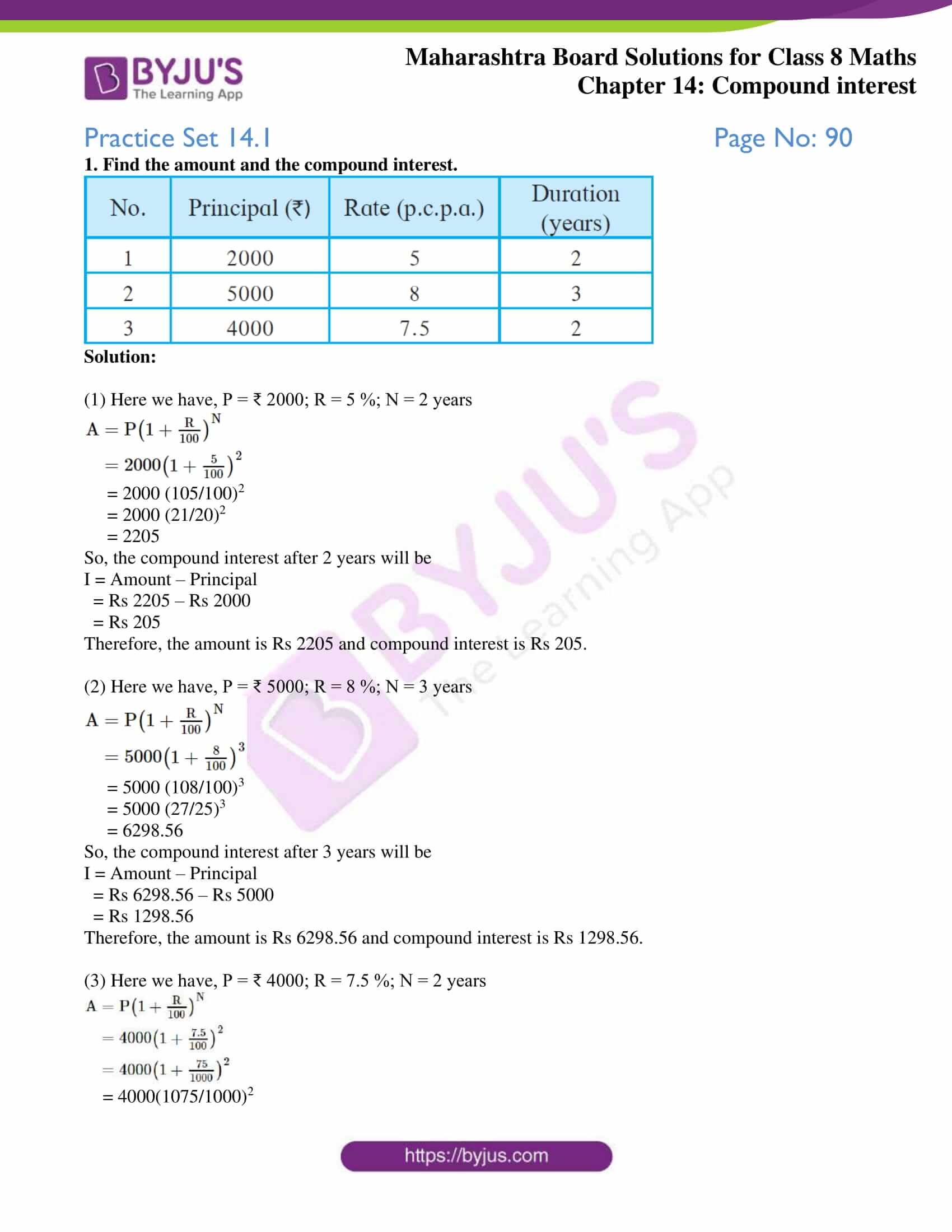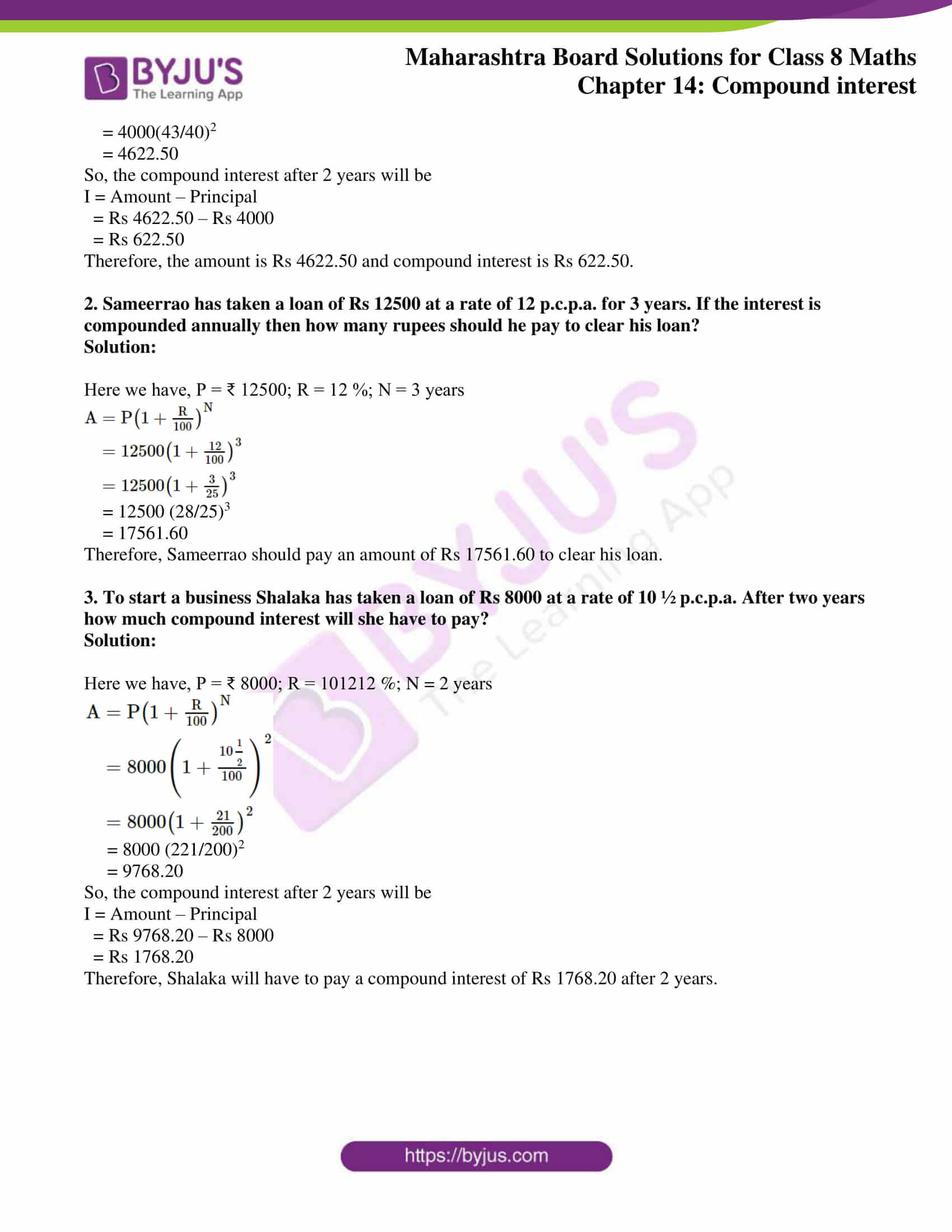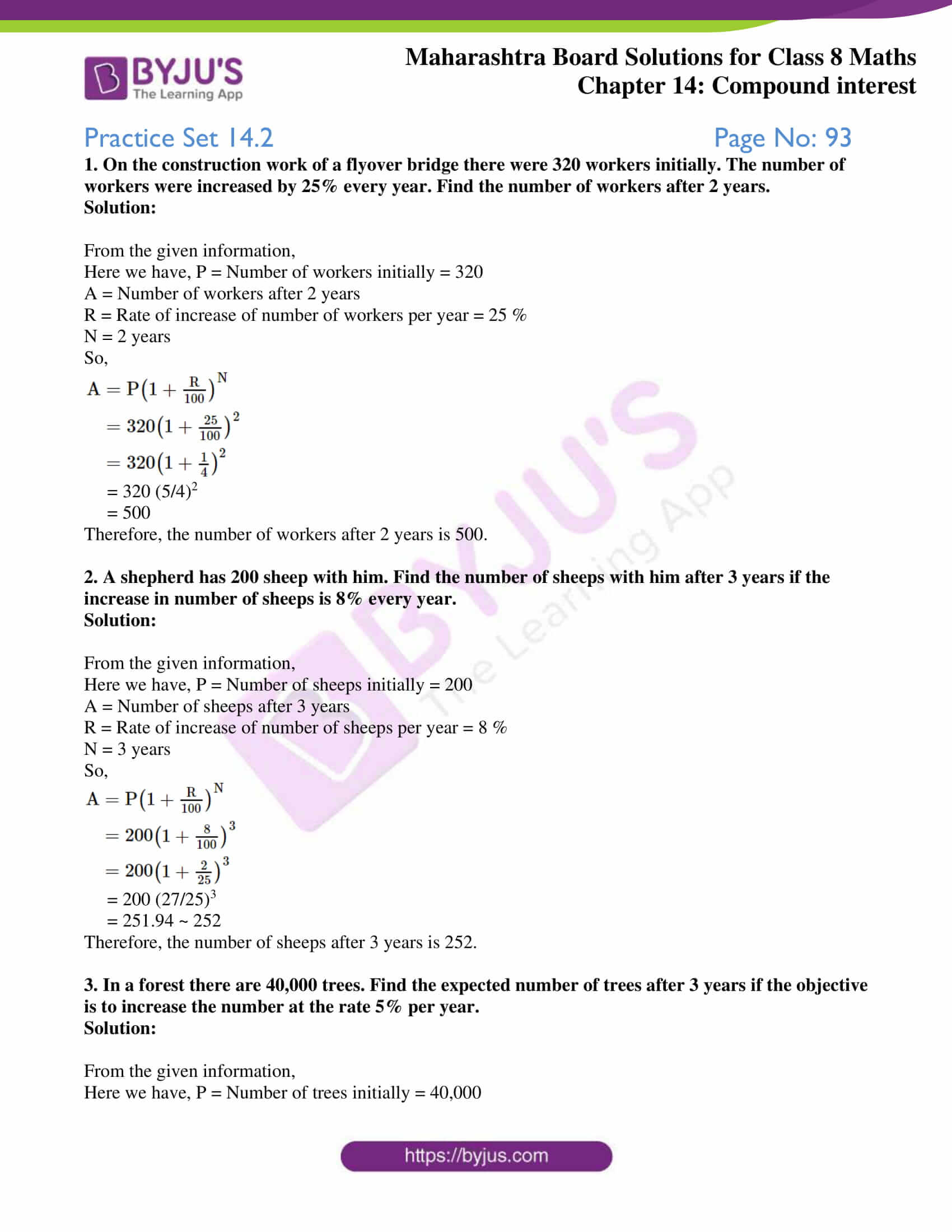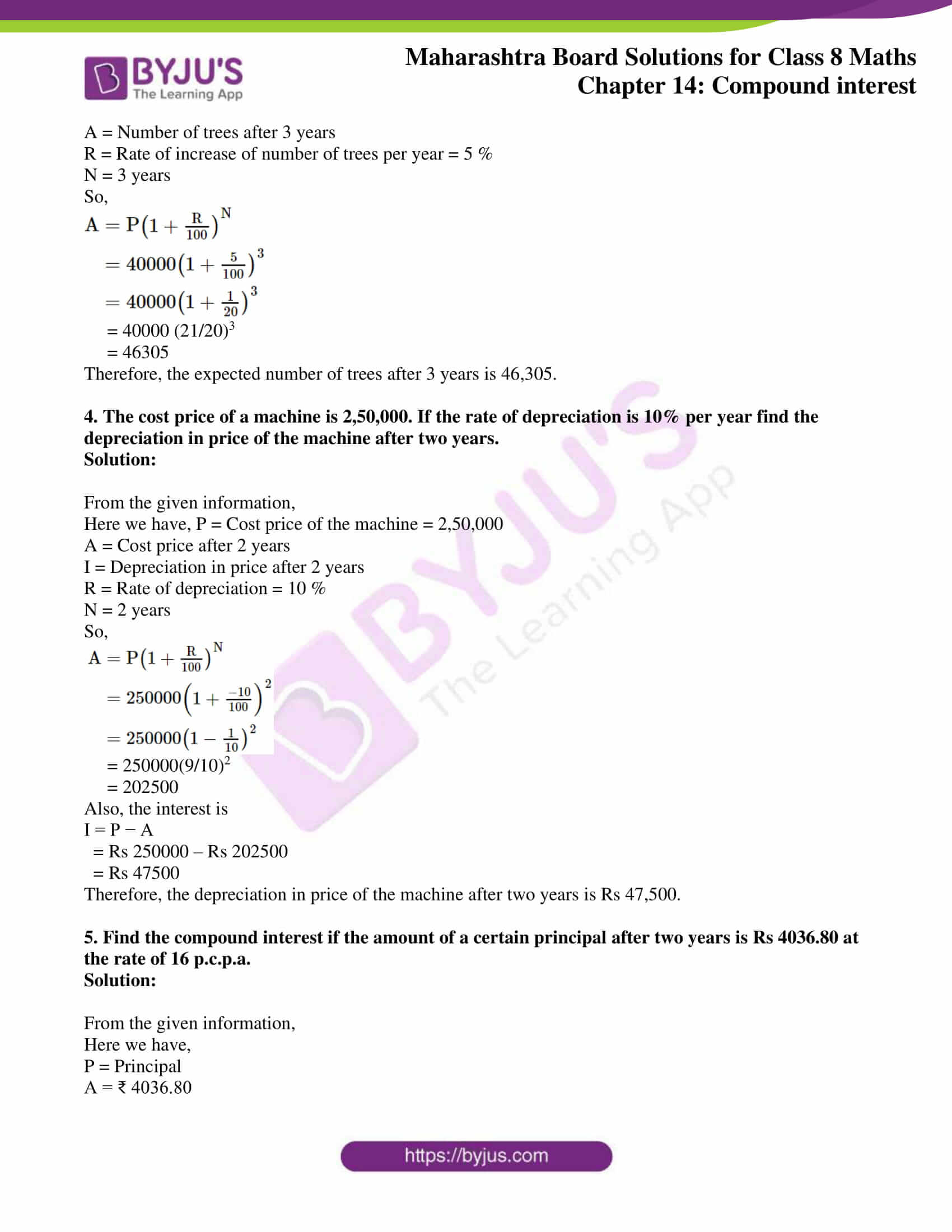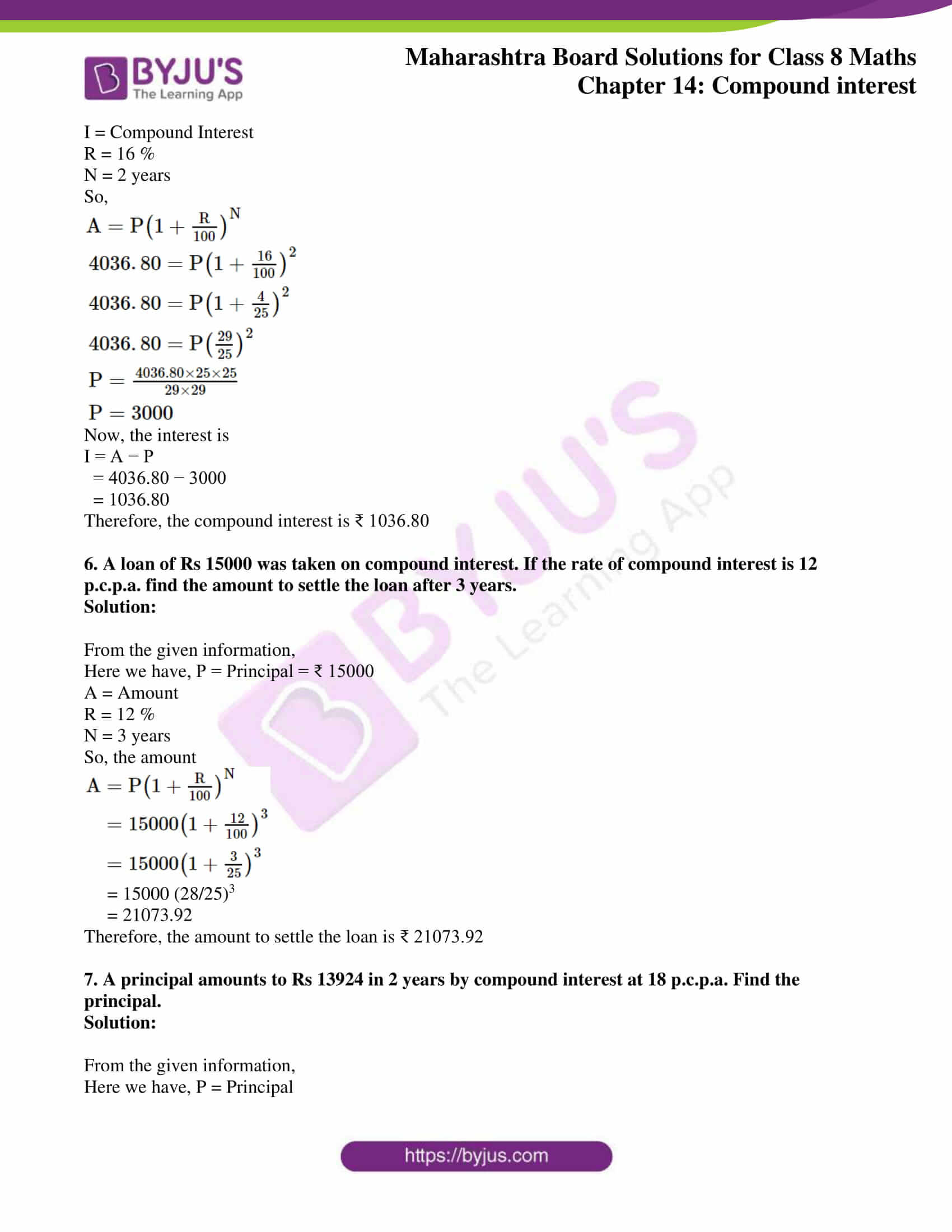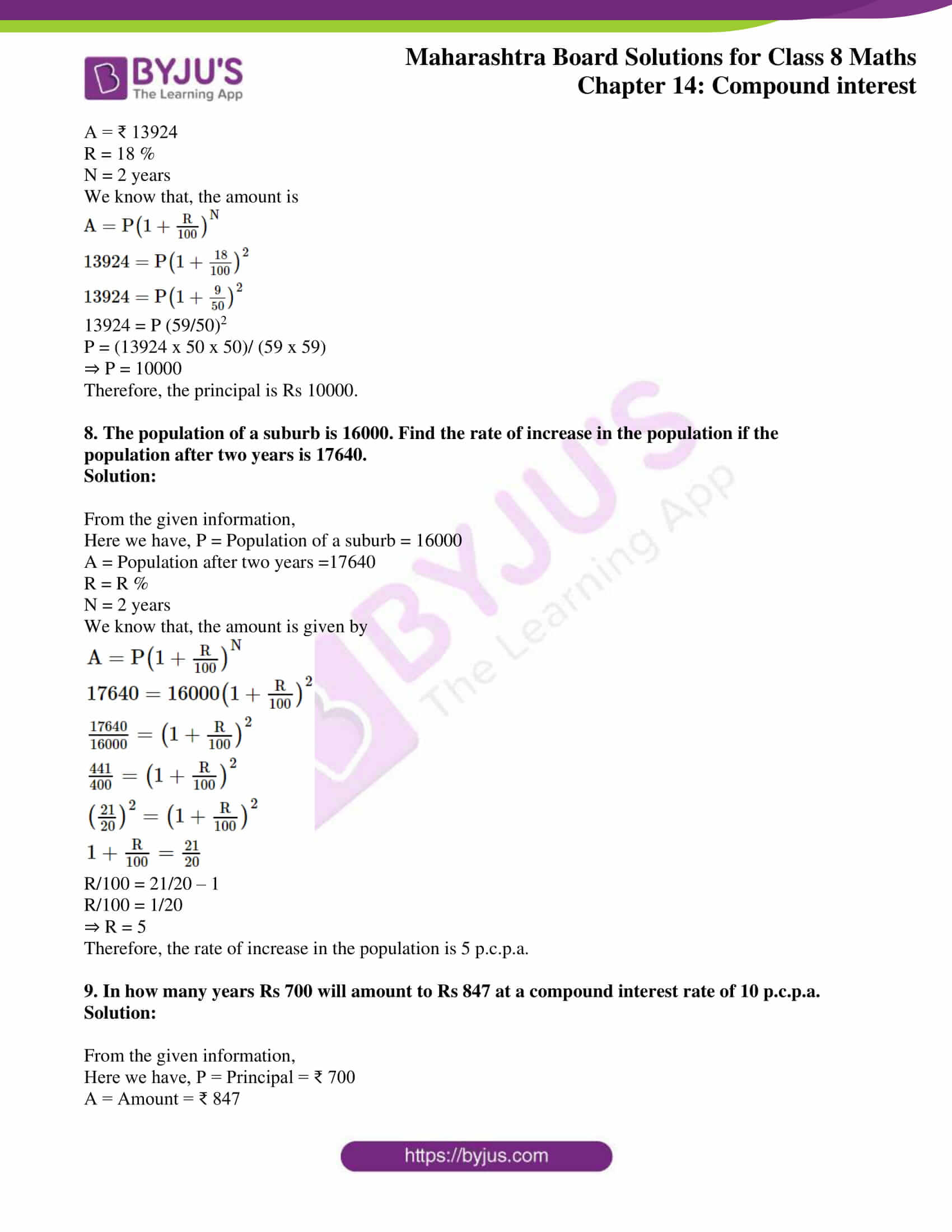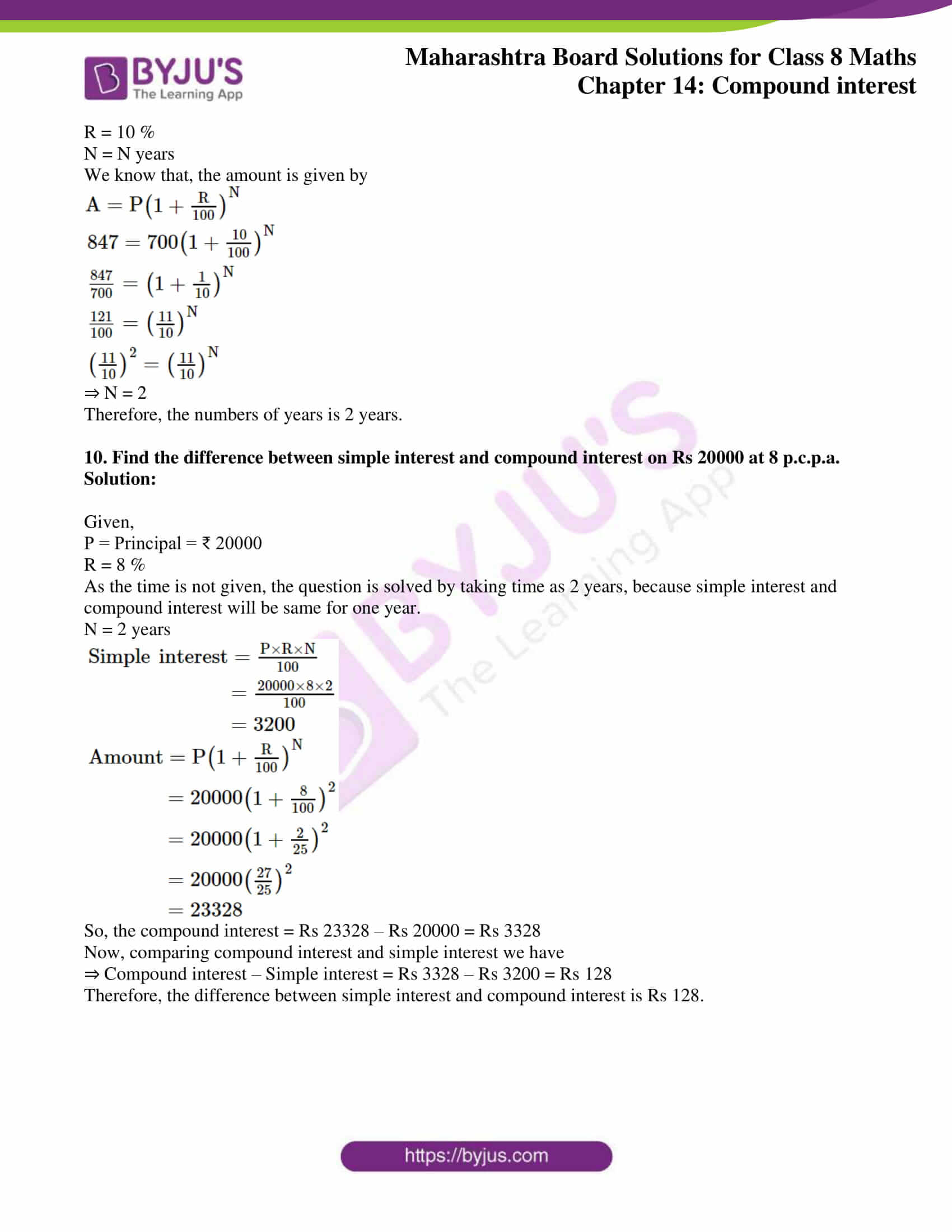### Access answers to Maharashtra Board Solutions For Class 8 Maths Part 2 Chapter 14 Compound interest.

Practice Set 14.1 Page No: 90

1. Find the amount and the compound interest.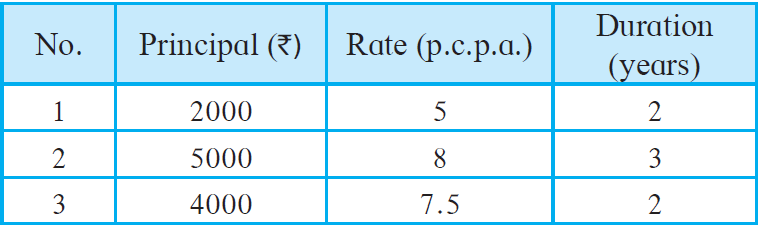Solution:

(1) Here we have, P = ₹ 2000; R = 5 %; N = 2 years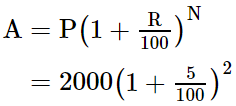= 2000 (105/100)2

= 2000 (21/20)2

= 2205

So, the compound interest after 2 years will be

I = Amount – Principal

= Rs 2205 – Rs 2000

= Rs 205

Therefore, the amount is Rs 2205 and compound interest is Rs 205.

(2) Here we have, P = ₹ 5000; R = 8 %; N = 3 years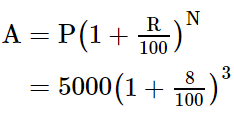= 5000 (108/100)3

= 5000 (27/25)3

= 6298.56

So, the compound interest after 3 years will be

I = Amount – Principal

= Rs 6298.56 – Rs 5000

= Rs 1298.56

Therefore, the amount is Rs 6298.56 and compound interest is Rs 1298.56.

(3) Here we have, P = ₹ 4000; R = 7.5 %; N = 2 years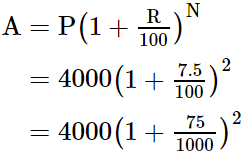= 4000(1075/1000)2

= 4000(43/40)2

= 4622.50

So, the compound interest after 2 years will be

I = Amount – Principal

= Rs 4622.50 – Rs 4000

= Rs 622.50

Therefore, the amount is Rs 4622.50 and compound interest is Rs 622.50.

2. Sameerrao has taken a loan of Rs 12500 at a rate of 12 p.c.p.a. for 3 years. If the interest is compounded annually then how many rupees should he pay to clear his loan?

Solution:

Here we have, P = ₹ 12500; R = 12 %; N = 3 years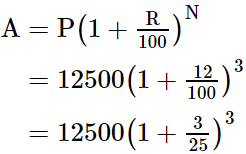= 12500 (28/25)3

= 17561.60

Therefore, Sameerrao should pay an amount of Rs 17561.60 to clear his loan.

3. To start a business Shalaka has taken a loan of Rs 8000 at a rate of 10 ½ p.c.p.a. After two years how much compound interest will she have to pay?

Solution:

Here we have, P = ₹ 8000; R = 101212 %; N = 2 years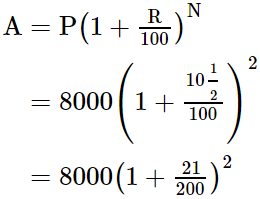= 8000 (221/200)2

= 9768.20

So, the compound interest after 2 years will be

I = Amount – Principal

= Rs 9768.20 – Rs 8000

= Rs 1768.20

Therefore, Shalaka will have to pay a compound interest of Rs 1768.20 after 2 years.

Practice Set 14.2 Page No: 93

1. On the construction work of a flyover bridge there were 320 workers initially. The number of workers were increased by 25% every year. Find the number of workers after 2 years.

Solution:

From the given information,

Here we have, P = Number of workers initially = 320

A = Number of workers after 2 years

R = Rate of increase of number of workers per year = 25 %

N = 2 years

So,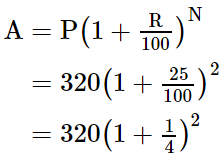= 320 (5/4)2

= 500

Therefore, the number of workers after 2 years is 500.

2. A shepherd has 200 sheep with him. Find the number of sheeps with him after 3 years if the increase in number of sheeps is 8% every year.

Solution:

From the given information,

Here we have, P = Number of sheeps initially = 200

A = Number of sheeps after 3 years

R = Rate of increase of number of sheeps per year = 8 %

N = 3 years

So,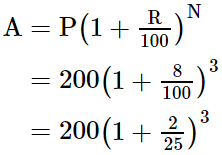= 200 (27/25)3

= 251.94 ~ 252

Therefore, the number of sheeps after 3 years is 252.

3. In a forest there are 40,000 trees. Find the expected number of trees after 3 years if the objective is to increase the number at the rate 5% per year.

Solution:

From the given information,

Here we have, P = Number of trees initially = 40,000

A = Number of trees after 3 years

R = Rate of increase of number of trees per year = 5 %

N = 3 years

So,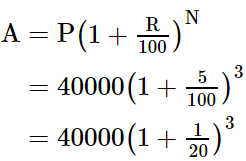= 40000 (21/20)3

= 46305

Therefore, the expected number of trees after 3 years is 46,305.

4. The cost price of a machine is 2,50,000. If the rate of depreciation is 10% per year find the depreciation in price of the machine after two years.

Solution:

From the given information,

Here we have, P = Cost price of the machine = 2,50,000

A = Cost price after 2 years

I = Depreciation in price after 2 years

R = Rate of depreciation = 10 %

N = 2 years

So,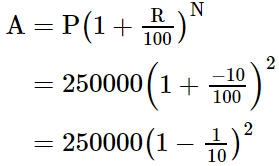= 250000(9/10)2

= 202500

Also, the interest is

I = P − A

= Rs 250000 – Rs 202500

= Rs 47500

Therefore, the depreciation in price of the machine after two years is Rs 47,500.

5. Find the compound interest if the amount of a certain principal after two years is Rs 4036.80 at the rate of 16 p.c.p.a.

Solution:

From the given information,

Here we have,

P = Principal

A = ₹ 4036.80

I = Compound Interest

R = 16 %

N = 2 years

So,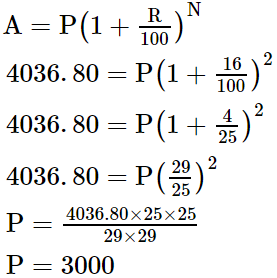Now, the interest is

I = A − P

= 4036.80 − 3000

= 1036.80

Therefore, the compound interest is ₹ 1036.80

6. A loan of Rs 15000 was taken on compound interest. If the rate of compound interest is 12 p.c.p.a. find the amount to settle the loan after 3 years.

Solution:

From the given information,

Here we have, P = Principal = ₹ 15000

A = Amount

R = 12 %

N = 3 years

So, the amount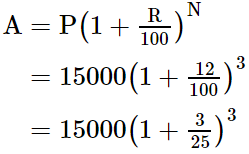= 15000 (28/25)3

= 21073.92

Therefore, the amount to settle the loan is ₹ 21073.92

7. A principal amounts to Rs 13924 in 2 years by compound interest at 18 p.c.p.a. Find the principal.

Solution:

From the given information,

Here we have, P = Principal

A = ₹ 13924

R = 18 %

N = 2 years

We know that, the amount is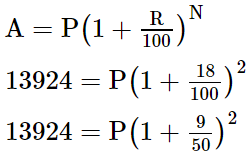13924 = P (59/50)2

P = (13924 x 50 x 50)/ (59 x 59)

⇒ P = 10000

Therefore, the principal is Rs 10000.

8. The population of a suburb is 16000. Find the rate of increase in the population if the population after two years is 17640.

Solution:

From the given information,

Here we have, P = Population of a suburb = 16000

A = Population after two years =17640

R = R %

N = 2 years

We know that, the amount is given by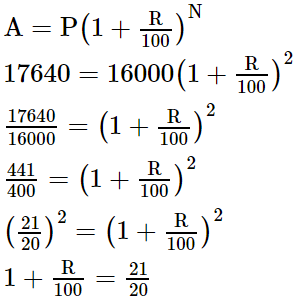R/100 = 21/20 – 1

R/100 = 1/20

⇒ R = 5

Therefore, the rate of increase in the population is 5 p.c.p.a.

9. In how many years Rs 700 will amount to Rs 847 at a compound interest rate of 10 p.c.p.a.

Solution:

From the given information,

Here we have, P = Principal = ₹ 700

A = Amount = ₹ 847

R = 10 %

N = N years

We know that, the amount is given by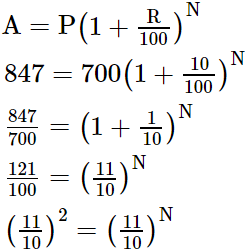⇒ N = 2

Therefore, the numbers of years is 2 years.

10. Find the difference between simple interest and compound interest on Rs 20000 at 8 p.c.p.a.

Solution:

Given,

P = Principal = ₹ 20000

R = 8 %

As the time is not given, the question is solved by taking time as 2 years, because simple interest and compound interest will be same for one year.

N = 2 years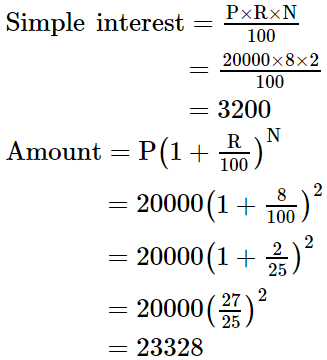So, the compound interest = Rs 23328 – Rs 20000 = Rs 3328

Now, comparing compound interest and simple interest we have

⇒ Compound interest – Simple interest = Rs 3328 – Rs 3200 = Rs 128

Therefore, the difference between simple interest and compound interest is Rs 128.

By referring to MSBSHSE Solutions, students will have a good hold of the concepts covered in this chapter. Chapter 14 Compound interest, enlightens students about compound interest and its calculations in their applications.

Solutions to the exercise problems are given for students to practice and secure excellent marks in their examination.

## Frequently Asked Questions on Maharashtra State Board Solutions for Class 8 Maths Chapter 14 Compound Interest

### Are these Maharashtra State Board Class 8 Maths Chapter 14 Solutions useful to prepare for the exams?

Yes, these Maharashtra State Board Class 8 Maths Chapter 14 Solutions are very helpful. Students are highly recommended practising these solutions after revising the subjects, as they set the basis for the questions that could get asked often in the board exams. By referring to these solutions after solving the questions will help the students to identify the mistakes and avoid them in the exams.